Home > CCA2 > Chapter 12 > Lesson 12.1.3 > Problem12-55

12-55.
1. Quickly sketch each of the following functions. Homework Help ✎

1. f(x) = (x − 1)2(x − 3)3(x − 5)2

2. g(x) = −(x − 1)2(x − 3)3(x − 5)2

3. Express g(x) in terms of f(x).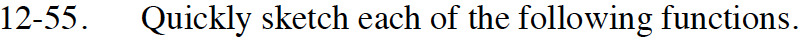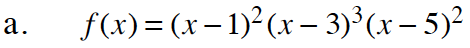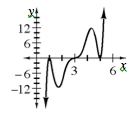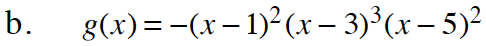How is this different from the function in part (a)?
What transformation is created by a negative sign?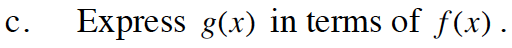Look at your graphs from parts (a) and (b).
What do they tell you about the relationship between the two functions?

g(x) = −f(x)